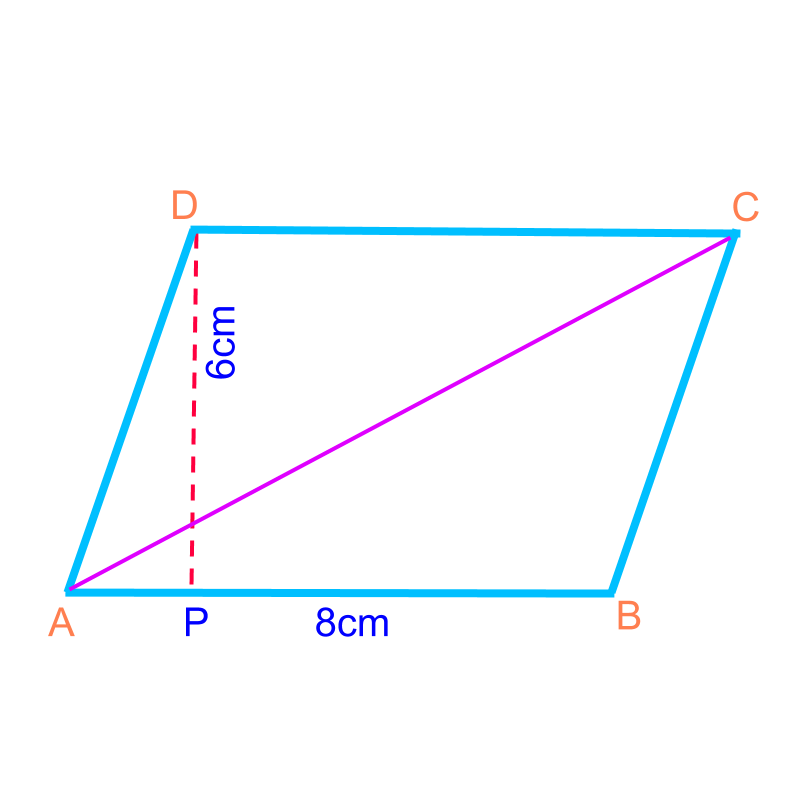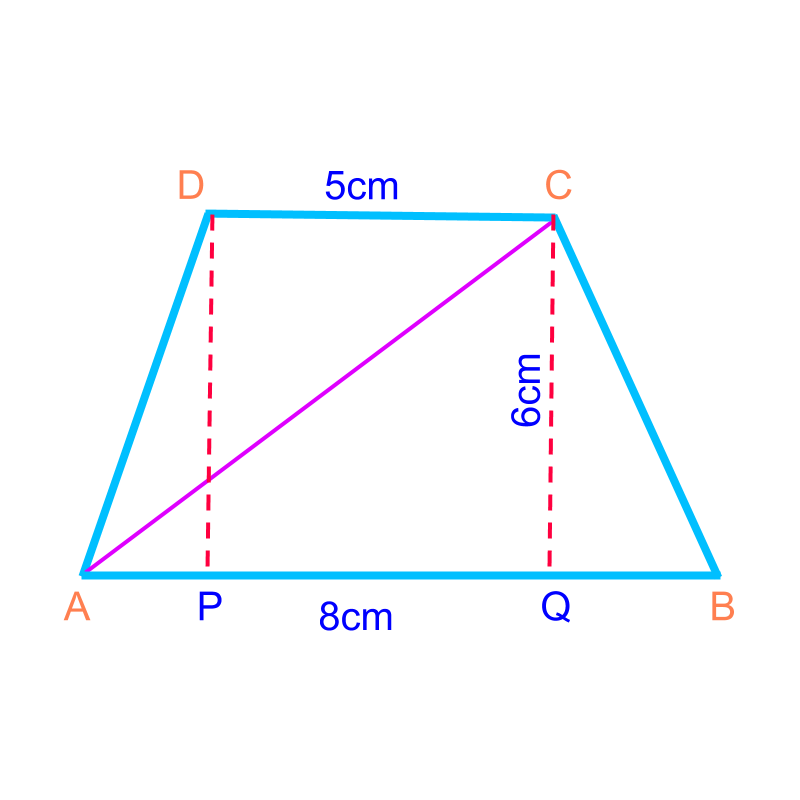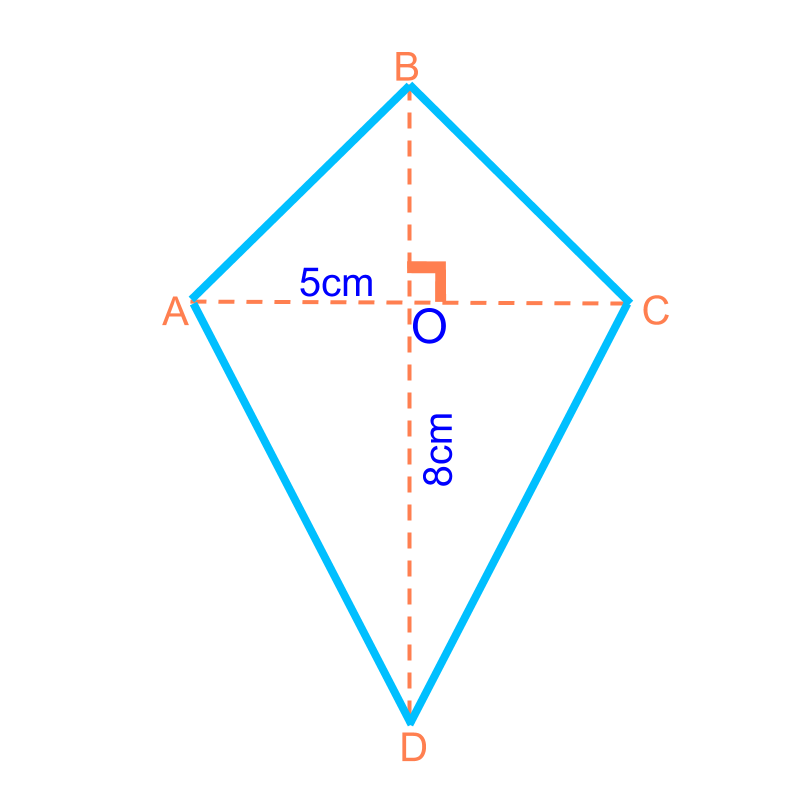maths > mensuration-basics

Perimeter and Area of Various Quadrilaterals

what you'll learn...

Overview

Area of Some Quadrilaterals : Consider the polygon shapes as combination of triangles and find sum of area of the triangles.$\phantom{\rule{1ex}{0ex}}\text{area of a parallelogram}\phantom{\rule{1ex}{0ex}}$$\textrm{a r e a o f a p a r a l \le \log r a m}$$=\phantom{\rule{1ex}{0ex}}\text{base}\phantom{\rule{1ex}{0ex}}×\phantom{\rule{1ex}{0ex}}\text{height}$$= \textrm{b a s e} \times \textrm{h e i g h t}$$\phantom{\rule{1ex}{0ex}}\text{area of a trapezium}\phantom{\rule{1ex}{0ex}}$$\textrm{a r e a o f a t r a p e z i u m}$$=\frac{1}{2}×\phantom{\rule{1ex}{0ex}}\text{sum of bases}\phantom{\rule{1ex}{0ex}}×\phantom{\rule{1ex}{0ex}}\text{height}$$= \frac{1}{2} \times \textrm{\sum o f b a s e s} \times \textrm{h e i g h t}$$\phantom{\rule{1ex}{0ex}}\text{area of a kite}\phantom{\rule{1ex}{0ex}}$$\textrm{a r e a o f a k i t e}$$=\frac{1}{2}×\phantom{\rule{1ex}{0ex}}\text{product of the diagonals}$$= \frac{1}{2} \times \textrm{\prod u c t o f t h e \mathrm{di} a g o n a l s}$

parallelogram

A parallelogram is a quadrilateral with two pairs of parallel sides.

Consider the parallelogram with base $8$$8$ cm and height $6$$6$ cm. The parallelogram is shown in the figure.

In general, to find area of polygons, consider them as combination of triangles. The same is applied to the parallelogram.The parallelogram $ABCD$$A B C D$ is considered to be two triangles $△ABC$$\triangle A B C$ and $△ACD$$\triangle A C D$.

The area of parallelogram

$=$$=$ sum of area of the two triangles

$=\frac{1}{2}×\overline{AB}×\overline{PD}+\frac{1}{2}\overline{CD}×\overline{PD}$$= \frac{1}{2} \times \overline{A B} \times \overline{P D} + \frac{1}{2} \overline{C D} \times \overline{P D}$

in a parallelogram the opposite sides are equal $\overline{AB}=\overline{CD}$$\overline{A B} = \overline{C D}$
$=\overline{AB}×\overline{PD}$$= \overline{A B} \times \overline{P D}$

$\phantom{\rule{1ex}{0ex}}\text{area}\phantom{\rule{1ex}{0ex}}=\phantom{\rule{1ex}{0ex}}\text{base}\phantom{\rule{1ex}{0ex}}×\phantom{\rule{1ex}{0ex}}\text{height}$$\textrm{a r e a} = \textrm{b a s e} \times \textrm{h e i g h t}$

trapezium

A trapezium is "a quadrilateral with one pair of parallel sides"

Consider the trapezium with two bases $8$$8$ cm & $5$$5$ cm, and height $6$$6$ cm. The trapezium is shown in the figure.The trapezium $ABCD$$A B C D$ is considered as two triangles $△ABC$$\triangle A B C$ and $△ACD$$\triangle A C D$.

The area of trapezium

$=$$=$ sum of area of the two triangles

$=\frac{1}{2}×\overline{AB}×\overline{PD}+\frac{1}{2}\overline{CD}×\overline{PD}$$= \frac{1}{2} \times \overline{A B} \times \overline{P D} + \frac{1}{2} \overline{C D} \times \overline{P D}$

$=\frac{1}{2}×\left(\overline{AB}+\overline{CD}\right)×\overline{PD}$$= \frac{1}{2} \times \left(\overline{A B} + \overline{C D}\right) \times \overline{P D}$

$\phantom{\rule{1ex}{0ex}}\text{area}\phantom{\rule{1ex}{0ex}}$$\textrm{a r e a}$$=\frac{1}{2}×\phantom{\rule{1ex}{0ex}}\text{sum of bases}\phantom{\rule{1ex}{0ex}}×\phantom{\rule{1ex}{0ex}}\text{height}$$= \frac{1}{2} \times \textrm{\sum o f b a s e s} \times \textrm{h e i g h t}$

kite

A kite is "a quadrilateral with two pair of equal and adjacent sides"

Consider the kite with two diagonals $8$$8$ cm and height $5$$5$ cm. The kite is shown in the figure.The kite $ABCD$$A B C D$ is considered to be two triangles $△BDA$$\triangle B D A$ and $△BDC$$\triangle B D C$.

The area of kite

$=$$=$ sum of area of the two triangles

$=\frac{1}{2}×\overline{BD}×\overline{AO}+\frac{1}{2}\overline{BD}×\overline{OC}$$= \frac{1}{2} \times \overline{B D} \times \overline{A O} + \frac{1}{2} \overline{B D} \times \overline{O C}$

$=\frac{1}{2}×\overline{BD}×\left(\overline{AO}+\overline{OC}\right)$$= \frac{1}{2} \times \overline{B D} \times \left(\overline{A O} + \overline{O C}\right)$

$=\frac{1}{2}×\overline{BD}×\overline{AC}$$= \frac{1}{2} \times \overline{B D} \times \overline{A C}$

$\phantom{\rule{1ex}{0ex}}\text{area}\phantom{\rule{1ex}{0ex}}=\frac{1}{2}×\phantom{\rule{1ex}{0ex}}\text{product of the diagonals}$$\textrm{a r e a} = \frac{1}{2} \times \textrm{\prod u c t o f t h e \mathrm{di} a g o n a l s}$

What is the area of a parallelogram of $2$$2$ cm length and $4$$4$ cm height?
The answer is "$8c{m}^{2}$$8 c {m}^{2}$".

summary

Area of Some Quadrilaterals : Consider the polygon shapes as combination of triangles and find sum of area of the triangles.$\phantom{\rule{1ex}{0ex}}\text{area of a parallelogram}\phantom{\rule{1ex}{0ex}}$$\textrm{a r e a o f a p a r a l \le \log r a m}$$=\phantom{\rule{1ex}{0ex}}\text{base}\phantom{\rule{1ex}{0ex}}×\phantom{\rule{1ex}{0ex}}\text{height}$$= \textrm{b a s e} \times \textrm{h e i g h t}$$\phantom{\rule{1ex}{0ex}}\text{area of a trapezium}\phantom{\rule{1ex}{0ex}}$$\textrm{a r e a o f a t r a p e z i u m}$$=\frac{1}{2}×\phantom{\rule{1ex}{0ex}}\text{sum of bases}\phantom{\rule{1ex}{0ex}}×\phantom{\rule{1ex}{0ex}}\text{height}$$= \frac{1}{2} \times \textrm{\sum o f b a s e s} \times \textrm{h e i g h t}$$\phantom{\rule{1ex}{0ex}}\text{area of a kite}\phantom{\rule{1ex}{0ex}}$$\textrm{a r e a o f a k i t e}$$=\frac{1}{2}×\phantom{\rule{1ex}{0ex}}\text{product of the diagonals}$$= \frac{1}{2} \times \textrm{\prod u c t o f t h e \mathrm{di} a g o n a l s}$

Outline

The outline of material to learn "Mensuration basics : Length, Area, & Volume" is as follows.

•  Measuring Basics

→   Introduction to Standards

→   Measuring Length

→   Accurate & Approximate Meaures

→   Measuring Area

→   Measuring Volume

→   Conversion between Units of Measure

•  2D shapes

→   Perimeter of Polygons

→   Area of Square & rectangle

→   Area of Triangle

→   Area of Polygons

→   Perimeter and area of a Circle

→   Perimeter & Area of Quadrilaterals

•  3D shapes

→   Surface Area of Cube, Cuboid, Cylinder

→   Volume of Cube, Cuboid, Cylinder